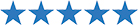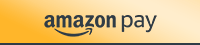# Slope, Graphing Linear Equations, & X and Y Intercepts Quiz

\$1.99

### Product Rating4.9
29 Ratings

• Easy, 100% Secure Checkout withCategory:

## Description

Do you need a slope, graphing linear equations, & x and y intercepts quiz for your middle school math class?

We did the hard work for you!

Slope, Graphing Linear Equations, & X- and Y- Intercepts Quiz

• 12 Questions

Topic Break Down:

1. Find the x- and y- intercepts given an equation.

2-5. Find the slope of the line, and find the intercepts.

6-8. Find the slope of the line given two points

9-10. Graph the equation given the slope and y-intercept

11-12. Graph the equation given a linear equation in slope-intercept form.

Please Note: The graphs print and copy well. The lines are dark and clear. The thumbnails do not do them justice.## Written by Bradley Augsburger (The Husband)

I support Amy behind the scenes. I'm the Dolce to her Gabbana.